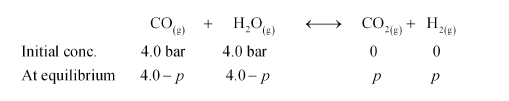# Dihydrogen gas used in Haber’s process is produced by reacting methane from natural gas with high temperature steam.`
Question:

Dihydrogen gas used in Haber’s process is produced by reacting methane from natural gas with high temperature steam. The first stage of two stage reaction involves the formation of CO and H2. In second stage, CO formed in first stage is reacted with more steam in water gas shift reaction,

$\mathrm{CO}(\mathrm{g})+\mathrm{H}_{2} \mathrm{O}(\mathrm{g}) \longleftrightarrow \mathrm{CO}_{2}(\mathrm{~g})+\mathrm{H}_{2}(\mathrm{~g})$

If a reaction vessel at $400^{\circ} \mathrm{C}$ is charged with an equimolar mixture of $\mathrm{CO}$ and steam such that $p_{\mathrm{co}}=p_{\mathrm{H}_{\mathrm{s}}, \mathrm{o}}=4.0$ bar, what will be the partial pressure of $\mathrm{H}_{2}$ at equilibrium? $\mathrm{Kp}=$ $10.1$ at $400^{\circ} \mathrm{C}$

Solution:

Let the partial pressure of both carbon dioxide and hydrogen gas be p. The given reaction is:It is given that $K_{\mathrm{p}}=10.1$.

Now,

$\frac{p_{\mathrm{CO}_{2}} \times p_{\mathrm{H}_{2}}}{p_{\mathrm{COH} \times} p_{2}}=K_{\mathrm{P}}$

$\Rightarrow \frac{p \times p}{(4.0-p)(4.0-p)}=10.1$

$\Rightarrow \frac{p}{4.0-p}=3.178$

$\Rightarrow p=12.712-3.178 p$

$\Rightarrow 4.178 p=12.712$

$\Rightarrow p=3.04$

Hence, at equilibrium, the partial pressure of $\mathrm{H}_{2}$ will be $3.04$ bar.